Report - Investigating Key Challenges in Applying Deep Learning Techniques to Integrated Computational Material Engineering

Authors: Ruijin Cang, Hechao Li, Houpu Yao, Yang Jiao, Yi Ren

1. Summary

We discuss two key challenges in applying machine learning techniques to computational microstructure-mediated material design: The first is related to improving model performance under small data (due to high acquisition cost of material data), and the second is on measuring model interpretability.

Our preliminary results lead to the following conclusions:

1. It is feasible to improve model performance under small data, by incorporating physical constraints to the model.
2. Even when a predictive structure-property model has high accuracy, it may still have misleading gradient information and is thus inappropriate for gradient-based microstructure design, i.e., high prediction accuracy does not guarantee interpretability.
3. One potential solution to 2 is to compute gradients of the property with respect to the latent space that defines the distribution of microstructures, projecting the incorrect gradient direction back into the domain of feasible microstructures. This can be achieved by learning a generative model.
4. We show that the solution in 3 leads to meaningful optimization of a sandstone microstructure: Along the direction (in the latent space) that increases the Young’s modulus, pore sizes decrease.

2. Introduction

While machine learning has shown promise in accelerating material design, two key concerns have been brought up repeatedly in recent discussions: (1) How useful would machine learning models (discriminative and generative) be when data is limited? And (2), are these models interpretable? More specifically, do models acquire physically meaningful traits (I use the word “trait” for models and reserve “property” for materials) consistent with physics-based theories? These questions are intriguing for both the machine learning and the material science communities, as they touch on the fundamental machine learning challenges with data efficiency and model interpretability, and are the current barrier for wider adoption of machine learning techniques to material design.

The first concern is motivated by the fact that often times only small data (e.g., measurements of processing settings, microstructures, and properties) is available or can be acquired for the design of a new material. From a machine learning perspective, the challenge is with fitting highly nonlinear functions that require complex models, while only a small amount of data is available. The second concern is rooted from the material community’s concern that “curve fitting” may not guarantee models with the correct physical meaning or feasible design rules. For example, the Hashin-Shtrikman bound defines the theoretical relationship between volume fraction and elastic modulus. Will a machine learning model be able to capture this relationship?

While we have not yet fully addressed these concerns, we report recent studies that point to tangible research directions towards this goal:

• The first study was an attempt to address the small data issue by exploiting hidden information in the data set. Specifically, in the setting of material design, we consider building a predictive structure-property model to accelerate microstructure optimization, with a small amount of authentic microstructures and a physics-based structure-property mapping. We seek to enrich the data by learning a generative model that will create artificial microstructures with negligible computation cost. These microstructures and their properties will then improve the accuracy of the predictive model. The issue, however, is that learning the generative model under small data is by itself challenging. We address this challenge by introducing an additional morphology consistency constraint to the learning of the generative model, thus enforcing the artificial microstructures to acquire the same distribution of morphologies as the authentic ones. The proposed model significantly outperforms standard generative models (e.g., variational autoencoders and Markov random fields) with the same amount of microstructure samples. The outcomes suggested that incorporating material-specific and physically-defined consistency constraints may potentially improve machine learning models under small data.

• The second study concerns about the interpretability of predictive structure-property models. We show that for a predictive structure-property model with high prediction accuracy, it may still produce misleading gradient information and thus cannot be used for microstructure optimization. We then show that an alternative gradient with respect to the latent space induced by the generative model can produce search directions towards meaningful microstructures with locally optimized properties. This result suggests that the combined (generative + predictive) model has better physical interpretability. To quantify interpretability, we define a trait of a structure-property model as a quantifiable constraint satisfied by the model. The interpretability can be measured as the similarity between the trait of the model and that of a physics-based model. We demonstrate the interpretability of a sandstone-modulus model, and discuss how model interpretability can be improved through an augmented learning process.

3. Microstructure Generation with Small Data

We discuss in this section the challenge of learning a generative model with a small amount of data. Readers can find more technical details from our paper.

A generative model maps one probability distribution defined on a (usually) low dimensional input space to another distribution in a (usually) high dimensional output space. Given a set of data points in the output space, the model is optimized to match its output distribution with the data distribution. Existing research on generative models focus on application domains where data is abundant. Yet with limited data, the generation quality of the state-of-the-art models is often far from desirable. For example, applying an autoencoder and a VAE to 200 sandstone microstructure samples will result in random generations shown in Fig. 1b and 1c, respectively. Similarly, the application of a Markov random field (MRF) model (another type of generative models, see Bostanabad et al. for example) results in artificial defects, as seen in Fig. 1d and 1f. The technical details and differences between VAE and MRF types of models can be found in this tutorial. In short, VAE indirectly matches the model and data distributions in the input space; while random fields directly characterize the data distribution in the output space. The former is often data demanding due to the potentially highly nonlinear mapping from inputs to outputs. While the latter partially addresses this challenge when a Markovian assumption is applicable (i.e., morphological patterns are local), this assumption does not hold for many material systems (where long-range spatial correlations exist).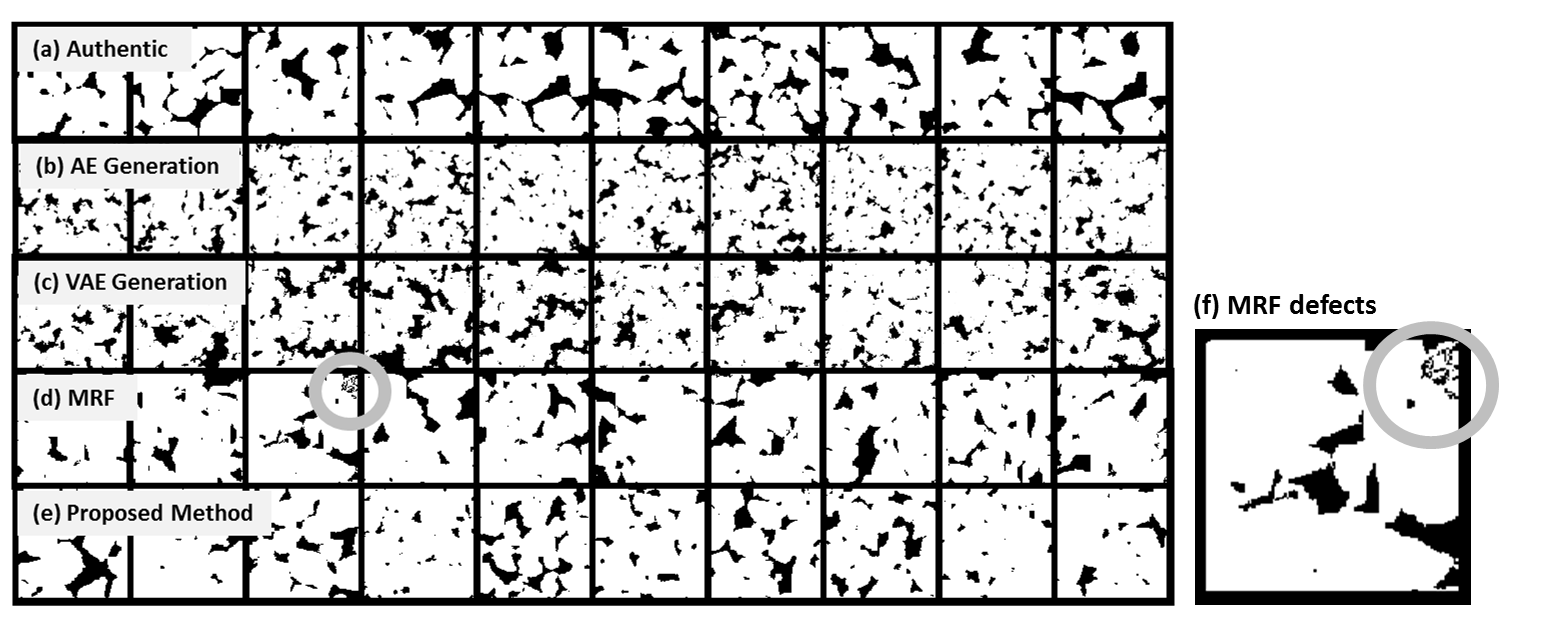Figure 1. (a) The authentic sandstone microstructure samples (b) Generations from a standard autoencoder (c) Generations from a standard VAE (d) Generations from the MRF method in Bostanabad et al. (e) Generations from our VAE+morphology method (f) An enlarged example of MRF generation with artificial defects

3.1 Morphologically consistent generative model

Our solution to this challenge is to introduce an additional morphology constraint to the training of the generative model. Specifically, we require the model to not only produce the correct distribution in the microstructure space, but also in a morphology space. We define morphology as the style vector of a microstructure (see Gatys et al.), and train the model so that its generations have smoothly changing morphologies consistent with the data. A comparison between the architectures of the autoencoder, VAE, and the proposed model can be found in Fig. 2-3. Results from the proposed model on sandstones are shown in Fig. 1e.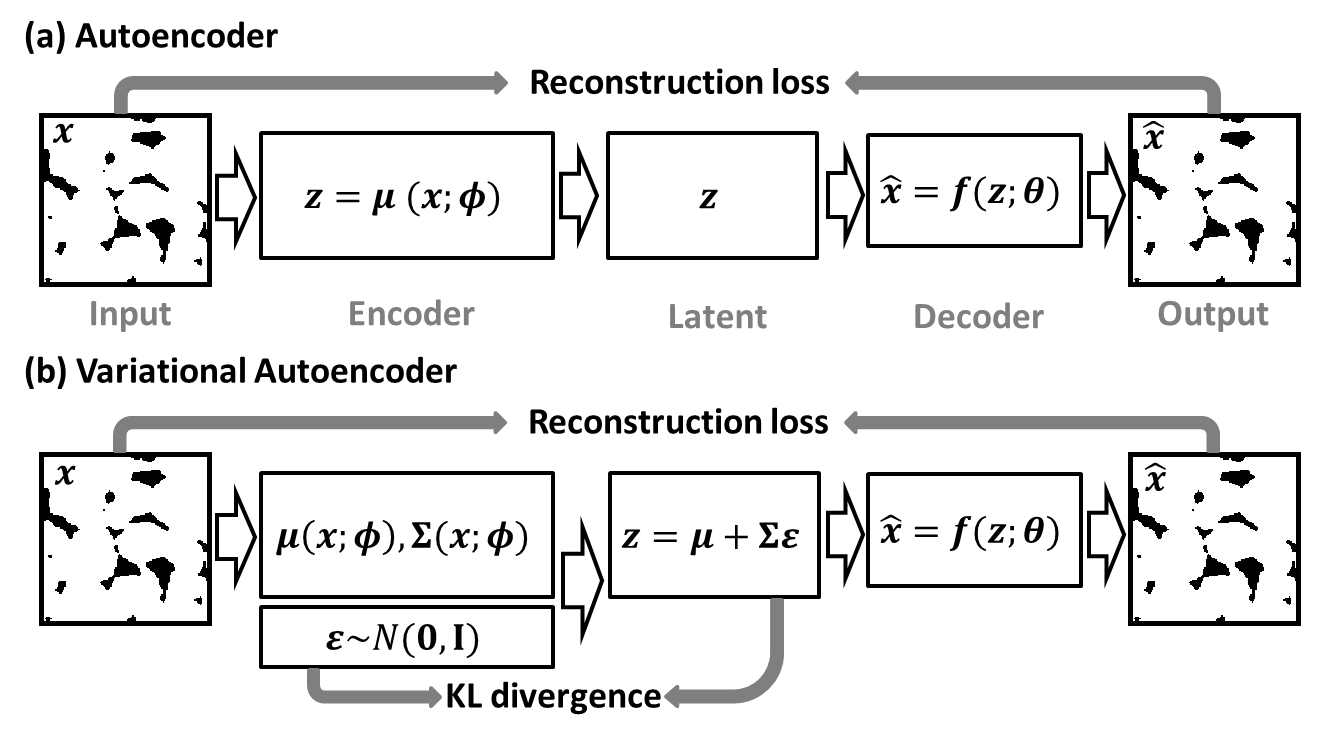Figure 2. Schematics for (a) an autoencoder and (b) a variational autoencoder.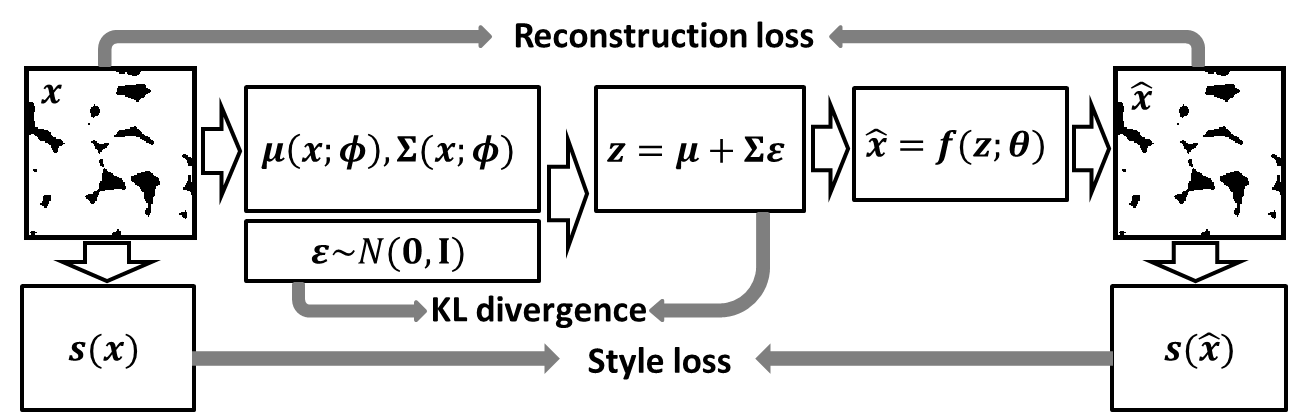Figure 3. Schematic for the proposed variational autoencoder with a morphology constraint.

3.2 Application of the generative model

We show in the following that the proposed generative model can produce additional training data that are valuable to the predictive structure-property model. We first derive baseline models for three mappings, from sandstone microstructures to Young’s modulus, diffusivity, and permeability, using 100 data points (microstructure-property pairs) for training and another 100 for validation (to prevent overfitting). The baseline prediction performance is evaluated using a third set of 100 test data points.

Two sets of artificial microstructures, 1000 samples in each, are created using the proposed method and the MRF, respectively. The true properties of these samples are computed. We then use these additional samples to improve the performance of the predictive models. Specifically, an increasing number of additional data points (from 50 to 1000) from the two sets are randomly added to the original training set to update the predictive model. We perform 10 independent random draws for each size of the additional data, and report the means and variances of the resultant test R-squares. The exception is with data size 1000, where all additional data points are used all together.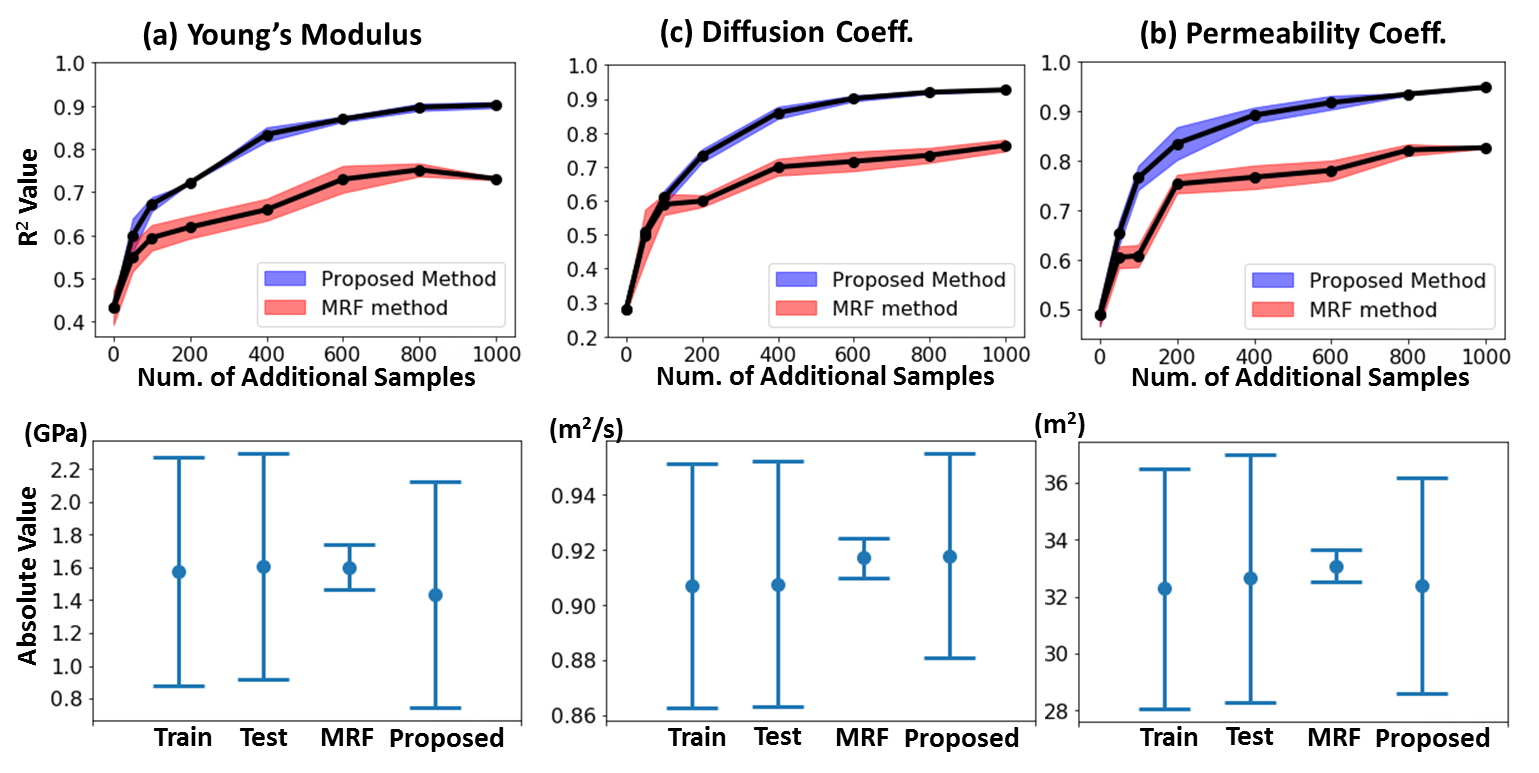Figure 4. (a) Property distributions of microstructures generated from the proposed and the MRF models. (b) Test R-square values at an increasing number of additional data points

Results are presented in Fig. 4 and summarized as follows. (1) Microstructures generated from the proposed method have property distributions similar to those of the authentic samples, while those generated from MRF have significantly smaller variances. We believe that this is because of the lack of variance in the volume fraction from the MRF generations (In Bostanabad et al., a model parameter is tuned so that all generations have the same volume fraction). It is worth noting that while a modified MRF model may resolve this issue, our approach does not require human identification or design of such features in the first place, as morphology variances are directly learned through the model. (2) The statistical difference in properties observed in (1) may explain the significant gap in the prediction performance of the resultant structure-property models, as seen in Fig. 4b: By feeding the network data from one distribution while asking it to predict on those drawn from another, we may encounter deteriorated performance even with an increased training data size.

4. Interpretation of the Models

We now investigate if the predictive model, as described above, is physically meaningful. A more formal question is: If we apply gradient descent to the predictive model, can we arrive at meaningful microstructures as local optimal solutions? Answering this question is important, as the value of predictive models in a material design context is to provide inexpensive and informative surrogates for optimization.

Fig. 5 answers this question empirically. Here we demonstrate the comparison between the optimization in the microstructure space and that in the input (latent) space of the generative model. For the former, we calculate the gradient of Young’s modulus in the microstructure space based on the predictive structure-property model with the highest R-square value from Fig. 4. For the latter, we first attach the generative model (Fig. 3) to the same predictive model, and use chain rule to compute the gradient in the latent space. Result shows that (1) gradient in the microstructure space are not meaningful, as it introduces unrealistic perturbations of an authentic microstructure, while (2) gradients in the latent space produce visually meaningful morphing of microstructures.

The first finding indicates that even models with high prediction accuracy may still be inappropriate for design when gradient-based search is necessary (e.g., for high-dimensional problems). This critical issue with deep neural networks has been brought to attention recently, see this discussion for example. The second finding is encouraging, and suggests a potential solution to the gradient issue. Conceptually, the generative model constraints the variation of microstructures to the latent space, which contains a much smaller set of dimensions that govern the microstructure distribution, i.e., the Jacobian introduced by the generative model projects an potentially improper gradient back to the domain of feasible microstructures.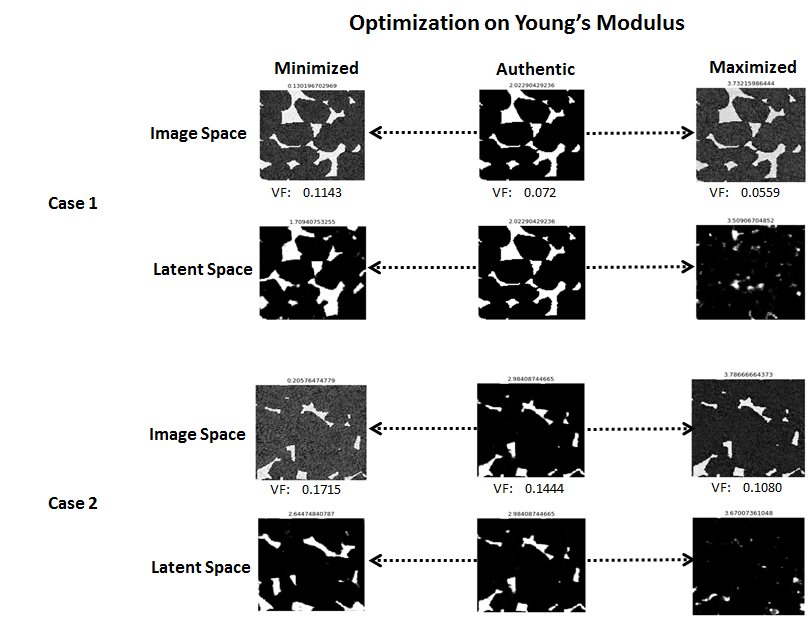Figure 5. Comparisons between Young’s modulus optimization in the microstructure space and the latent space using the best predictive model in Fig. 4 and the generative model in Fig. 3.

4.1 Model interpretability

The visual comparison in Fig. 5 motivates the seeking of a quantitative measure for comparing the goodness of property gradients. We call this measure model interpretability. One potential definition of this measure is as follows: We first define a trait of a structure-property model as a quantifiable constraint satisfied by the model (e.g., the Hashin-Shtrikman bound defines the relationship between volume fraction and elastic modulus). Model interpretability can then be defined as the similarity between its own trait and that of the physics-based (ground truth) model.

We are still conducting more experiments to validate this idea. An initial result in Fig. 6 illustrates the change in volume fraction along that of the Young’s modulus for the sandstone system. The results are derived using the combined generative+predictive model and from 100 independently drawn initial samples from the latent space. The negative gradient of the volume fraction indicates reduction of the pore phase along increasing Young’s modulus, a trait that is consistent with the physics-based model.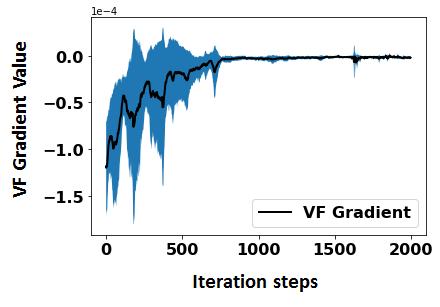Figure 6. The gradient of volume fraction along the optimization of the Young’s modulus with respect to the latent space of the sandstone system.

This result suggests that it is feasible to augment a predictive structure-property model by introducing an additional training loss on model interpretability. More concretely, we can define a differentiable loss using a set of known traits of the physics-based structure-property mapping, and incorporate the computation of these traits (which may involve symbolic derivatives of the predictive model) into the training process.